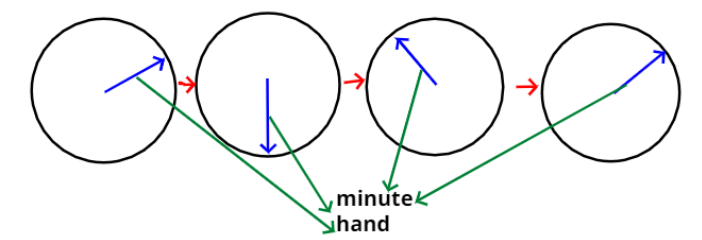Courses
Courses for Kids
Free study material
Free LIVE classes
MoreLIVE
Join Vedantu’s FREE Mastercalss

# The minute hand of a circular clock is 15cm long. How far does the tip of the minute hand moves in 1 hour? (Take $\pi = 3.14$)Verified
363.9k+ views
Hint:- Find circumference of clock.As, we are given the length of the minute hand of the clock.
And we can see from the above figure that tip of the minute hand forms the circle
When it finishes one complete round . i.e. In one hour.
And the radius of the circle will be the length of the minute hand.
So, distance made by the tip of minute hand in 1 hour = Circumference of the circle made
by the minute hand.
As, we know that the circumference of the circle is given as, $2\pi r$.
Where, r is the radius of the circle.
And here radius, r $= 15$cm.
So, circumference of the circle made will be,
$\Rightarrow 2*\pi *15$cm
$\Rightarrow 30\pi = 30*3.14 = 94.2$cm
Hence the tip of the minute hand moves 94.2cm in one hour.
Note:- Whenever we came up with this type of problem then remember that tip of any
The hand of a clock covers a distance of circumference of the circle made by that hand in one hour.
But if we are asked to find the area covered by that hand in one hour then it will be equal to
the area of the circle made by that hand.

Last updated date: 23rd Sep 2023
Total views: 363.9k
Views today: 9.63k